Back to Contents

# New Kinds of Data

So far, we have considered the simple types int, bool, char, the compound type list, and tuples. We have built functions from and to these types. It would be possible to encode anything we wanted as lists and tuples of these types, but it would lead to complex and error-strewn programs. It is time to make our own types. New types are introduced using `type`. Here’s a type for colours:

` OCaml`

`# type colour = Red | Green | Blue | Yellow;;`
`type colour = Red | Green | Blue | Yellow`

The name of our new type is colour. It has four constructors, written with an initial capital letter: `Red`, `Green`, `Blue`, and `Yellow`. These are the possible forms a value of type colour may take. Now we can build values of type colour: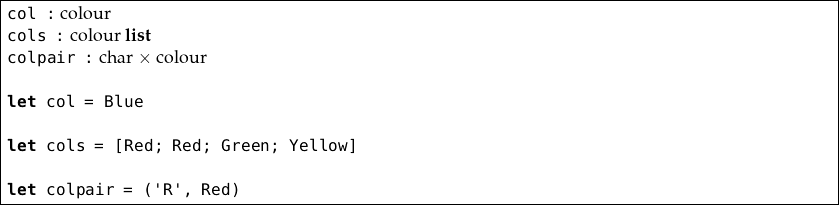Let us extend our type to include any other colour which can be expressed in the RGB (Red, Green, Blue) colour system (each component ranges from 0 to 255 inclusive, a standard range giving about 16 million different colours).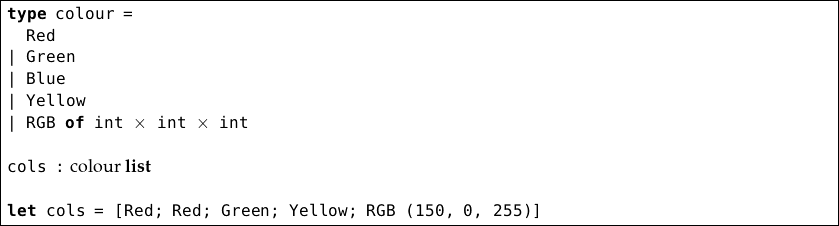We use `of` in our new constructor, to carry information along with values built with it. Here, we are using something of type int × int × int. Notice that the list `cols` of type colour list contains varying things, but they are all of the same type, as required by a list. We can write functions by pattern matching over our new type: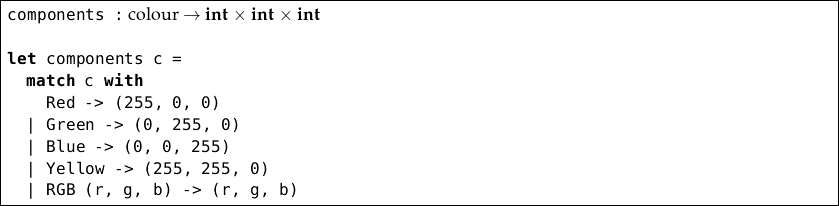Types may contain a type variable like α to allow the type of part of the new type to vary – i.e. for the type to be polymorphic. For example, here is a type used to hold either nothing, or something of any type:

` OCaml`

`# type 'a option = None | Some of 'a;;`
`type 'a option = None | Some of 'a`

We can read this as “a value of type α option is either nothing, or something of type α”. For example: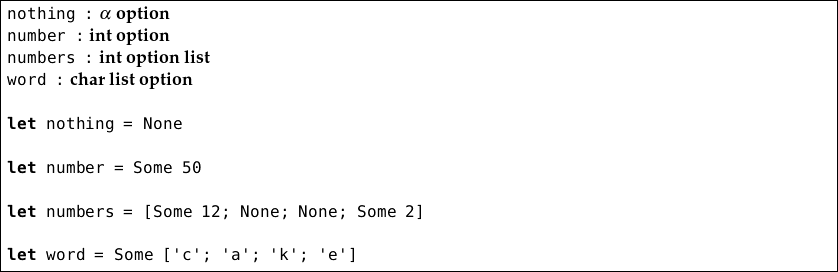The option type is useful as a more manageable alternative to exceptions where the lack of an answer is a common (rather than genuinely exceptional) occurrence. For example, here is a function to look up a value in a dictionary, returning `None` instead of raising an exception if the value is not found: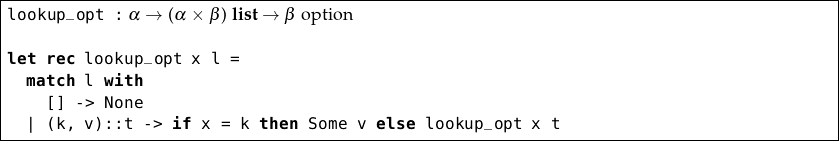Now, there is no need to worry about exception handling – we just pattern match on the result of the function.

In addition to being polymorphic, new types may also be recursively defined. We can use this functionality to define our own lists, just like the built-in lists in OCaml but without the special notation:

` OCaml`

`# type 'a sequence = Nil | Cons of 'a * 'a sequence;;`
`type 'a sequence = Nil | Cons of 'a * 'a sequence`

We have called our type sequence to avoid confusion. It has two constructors: `Nil` which is equivalent to `[]`, and `Cons` which is equivalent to the `::` operator. `Cons` carries two pieces of data with it – one of type α (the head) and one of type α sequence (the tail). This is the recursive part of our definition. Now we can make our own lists equivalent to OCaml’s built-in ones: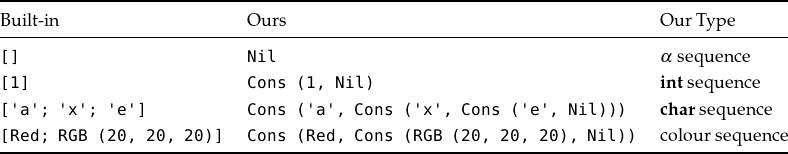Now you can see why getting at the last element of a list in OCaml is harder than getting at the first element – it is deeper in the structure. Let us compare some functions on OCaml lists with the same ones on our new sequence type. First, the ones for built-in lists.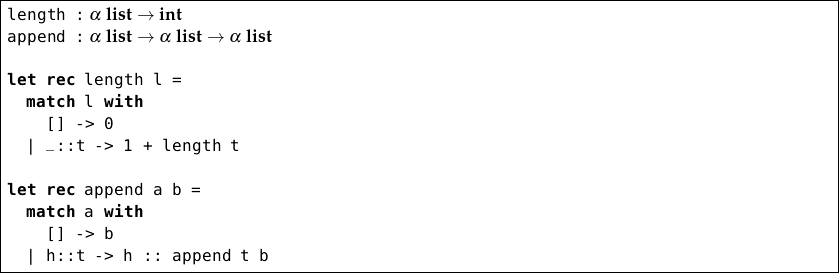And now the same functions with our new sequence type: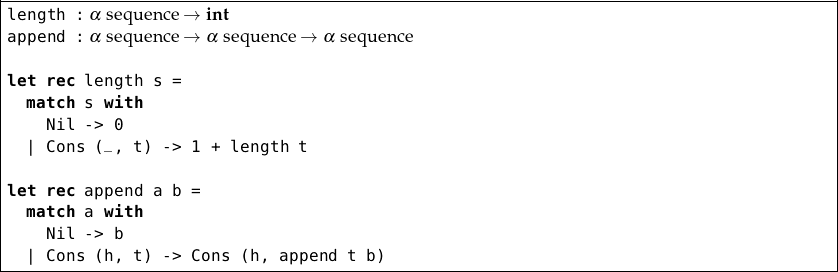Notice how all the conveniences of pattern matching such as completeness detection and the use of the underscore work for our own types too.

## A Type for Mathematical Expressions

Our sequence was an example of a recursively-defined type, which can be processed naturally by recursive functions. Mathematical expressions can be modeled in the same way. For example, the expression 1 + 2 × 3 could be drawn like this: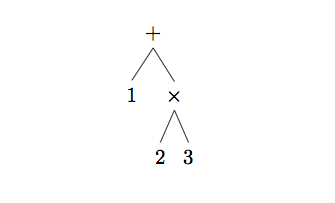Notice that, in this representation, we never need parentheses – the diagram is unambiguous. We can evaluate the expression by reducing each part in turn: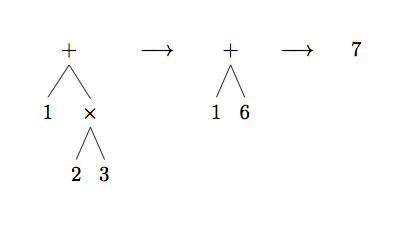Here’s a suitable type for such expressions: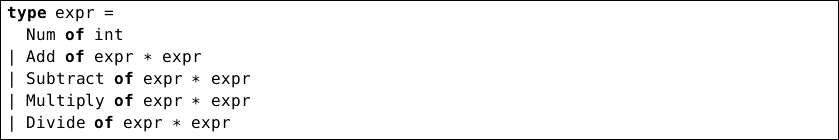For example, the expression 1 + 2 * 3 is represented in this data type as:

`Add (Num 1, Multiply (Num 2, Num 3))`

We can now write a function to evaluate expressions: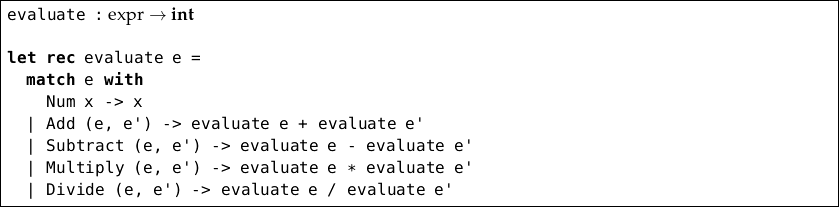Building our own types leads to clearer programs with more predictable behaviour, and helps us to think about a problem – often the functions are easy to write once we have decided on appropriate types.

## Questions

1. Design a new type rect for representing rectangles. Treat squares as a special case.

2. Now write a function of type rect int to calculate the area of a given rect.

3. Write a function which rotates a rect such that it is at least as tall as it is wide.

4. Use this function to write one which, given a rect list, returns another such list which has the smallest total width and whose members are sorted narrowest first.

5. Write `take`, `drop`, and `map` functions for the sequence type.

6. Extend the expr type and the `evaluate` function to allow raising a number to a power.

7. Use the option type to deal with the problem that `Division_by_zero` may be raised from the `evaluate` function.